Operation

Operation

「運算」。名聞天下、流芳百世的函數。因為大家經常使用這些函數，所以大家特地訂立數學符號、特地稱為運算。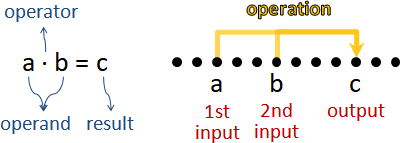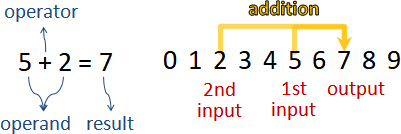Operation的輸入可以有許多個

Operation的輸出可以有許多個

Operation的輸入和輸出可以是不同類型

Operation的輸入們可以是不同類型

Group

Group

「群」是兩種東西合體：多個元素（一個集合）、一個運算。必須滿足四個規定。

```1. Closure           閉包：無論取誰運算，結果仍是那些元素。
a∙b = c
a∈S, b∈S ⇒ c∈S

2. Associativitiy    結合律：無論誰先運算，結果一致。
(a∙b)∙c = a∙(b∙c)

3. Identity          單位元素1：實施運算，結果不變。
a∙1 = 1∙a = a

4. Inverse           反元素a̅：實施運算，結果相消，得到單位元素。
a∙a̅ = a̅∙a = 1
```

```a b c：任意元素。
1 a̅：符合規定的某個元素（某些元素）。必須找到。
```

```S：這個群的元素們（集合）。
∙：這個群的運算。
=：等於。詳情請自行學習集合論。
∈：屬於。詳情請自行學習集合論。
,：與。詳情請自行學習集合論。
```

```集合：整數ℤ                ℤ = {..., -2, -1, 0, 1, 2, ...}

1. 2+3 = 5                 a = 2, b = 3, c = 5
2∈ℤ, 3∈ℤ ⇒ 5∈ℤ
2. (2+3)+4 = 2+(3+4)       a = 2, b = 3, c = 4
3. 2+0 = 0+2 = 2           a = 2, 1 = 0
4. 2+(-2) = (-2)+2 = 0     a = 2, a̅ = -2, 1 = 0
```

```集合：整數ℤ                ℤ = {..., -2, -1, 0, 1, 2, ...}

1. 2×3 = 6                 a = 2, b = 3, c = 6
2∈ℤ, 3∈ℤ ⇒ 6∈ℤ
2. (2×3)×4 = 2×(3×4)       a = 2, b = 3, c = 4
3. 2×1 = 1×2 = 2           a = 2, 1 = 1
4. 2×½ = ½×2 = 1           a = 2, a̅ = ½, 1 = 1
```

```集合：餘數ℤₙ                     ℤ₅ = {0, 1, 2, 3, 4}

1. 2+3 ≡ 0           (mod 5)     a = 2, b = 3, c = 0
2∈ℤ₅, 3∈ℤ₅ ⇒ 0∈ℤ₅
2. (2+3)+4 ≡ 2+(3+4) (mod 5)     a = 2, b = 3, c = 4
3. 2+0 ≡ 0+2 ≡ 2     (mod 5)     a = 2, 1 = 0
4. 2+3 ≡ 3+2 ≡ 0     (mod 5)     a = 2, a̅ = 3, 1 = 0
```

```集合：與模數互質的數字ℤₙˣ         ℤ₈ˣ = {1, 3, 5, 7}

1. 3×5 ≡ 7           (mod 8)     a = 3, b = 5, c = 7
3∈ℤ₈ˣ, 5∈ℤ₈ˣ ⇒ 7∈ℤ₈ˣ
2. (3×5)×7 ≡ 3×(5×7) (mod 8)     a = 3, b = 5, c = 7
3. 5×1 ≡ 1×5 ≡ 5     (mod 8)     a = 5, 1 = 1
4. 5×5 ≡ 5×5 ≡ 1     (mod 8)     a = 5, a̅ = 5, 1 = 1
```

```集合：實數向量ℝₙ

2. (a×b)×c ≠ a×(b×c)
```

Commutative Group（Abelian Group）

「交換群」。追加第五點規則。

```5. Commutativity     交換律：輸入交換順序，輸出仍然相同。
a∙b = b∙a
```

```集合：可逆矩陣

1. ⎡ 1 2 ⎤ ⎡ 2 2 ⎤ = ⎡  8 10 ⎤
⎣ 3 4 ⎦ ⎣ 3 4 ⎦   ⎣ 18 22 ⎦

⎡ 1 2 ⎤ ∈ ℝ₂ₓ₂  ⎡ 2 2 ⎤ ∈ ℝ₂ₓ₂ ⇒ ⎡  8 10 ⎤ ∈ ℝ₂ₓ₂
⎣ 3 4 ⎦       , ⎣ 3 4 ⎦          ⎣ 18 22 ⎦

a = ⎡ 1 2 ⎤   b = ⎡ 2 2 ⎤   c = ⎡  8 10 ⎤
⎣ 3 4 ⎦ ,     ⎣ 3 4 ⎦ ,     ⎣ 18 22 ⎦

2. ⎛ ⎡ 1 2 ⎤ ⎡ 2 2 ⎤ ⎞ ⎡ 3 2 ⎤ = ⎡ 1 2 ⎤ ⎛ ⎡ 2 2 ⎤ ⎡ 3 2 ⎤ ⎞
⎝ ⎣ 3 4 ⎦ ⎣ 3 4 ⎦ ⎠ ⎣ 3 4 ⎦   ⎣ 3 4 ⎦ ⎝ ⎣ 3 4 ⎦ ⎣ 3 4 ⎦ ⎠

a = ⎡ 1 2 ⎤ , b = ⎡ 2 2 ⎤ , c = ⎡ 3 2 ⎤
⎣ 3 4 ⎦       ⎣ 3 4 ⎦       ⎣ 3 4 ⎦

3. ⎡ 4 3 ⎤ ⎡ 1 0 ⎤ = ⎡ 1 0 ⎤ ⎡ 4 3 ⎤ = ⎡ 4 3 ⎤
⎣ 3 2 ⎦ ⎣ 0 1 ⎦   ⎣ 0 1 ⎦ ⎣ 3 2 ⎦   ⎣ 3 2 ⎦

a = ⎡ 4 3 ⎤ , 1 = ⎡ 1 0 ⎤
⎣ 3 2 ⎦       ⎣ 0 1 ⎦

4. ⎡ 4 3 ⎤ ⎡ -2 3 ⎤ = ⎡ -2 3 ⎤ ⎡ 4 3 ⎤ = ⎡ 1 0 ⎤
⎣ 3 2 ⎦ ⎣  3 4 ⎦   ⎣  3 4 ⎦ ⎣ 3 2 ⎦   ⎣ 0 1 ⎦

a = ⎡ 4 3 ⎤ , a̅ = ⎡ -2 3 ⎤ , 1 = ⎡ 1 0 ⎤
⎣ 3 2 ⎦       ⎣  3 4 ⎦       ⎣ 0 1 ⎦

5. ⎡ 1 2 ⎤ ⎡ 2 2 ⎤ ≠ ⎡ 2 2 ⎤ ⎡ 1 2 ⎤
⎣ 3 4 ⎦ ⎣ 3 4 ⎦   ⎣ 3 4 ⎦ ⎣ 3 4 ⎦

a = ⎡ 1 2 ⎤ , b = ⎡ 2 2 ⎤
⎣ 3 4 ⎦       ⎣ 3 4 ⎦
```

```集合：三維旋轉矩陣（正規正交矩陣，determinant是1）

```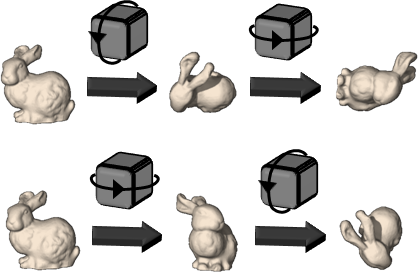Order

「秩」。群的大小。元素數量。集合大小。

Generating Set

「生成集合」。取出一些元素。以這些元素及其反元素，實施一長串運算，例如a∙b∙a̅∙b∙b∙b，可以得到每一種元素。

Subgroup

「子群」。取出一些元素。以這些元素及其反元素，實施一長串運算，還是只能得到這些元素。

Lagrange's Theorem：子群的大小，整除群的大小。

Cauchy's Theorem：當質數p整除群的大小（例如排列群），那麼此群存在一個元素g（例如一個排列），使得 g^p = 1（此排列套用p次，每個軌道剛好走零步）。

Cayley's Theorem：隨便一個群，一定可以等價地變成某個對稱群的子群（例如排列群）。

Cyclic Group

Cyclic Group

「循環群」。生成集合，大小可以是一。想要生成每一種元素，只需一個元素，無需一組元素。

```g
g∙g
g∙g∙g
g∙g∙g∙g
:
```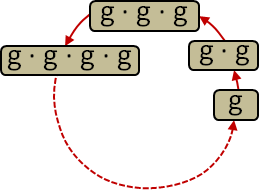Generator

「生成子」。取出一個元素。以這個元素（及其反元素），實施一長串運算，例如g∙g∙g∙g∙g，可以得到每一種元素。

```集合：餘數ℤ₈                ℤ₈ = {0, 1, 2, 3, 4, 5, 6, 7}

g ≡ 3 (mod 8)
g+g ≡ 6 (mod 8)
g+g+g ≡ 1 (mod 8)
g+g+g+g ≡ 4 (mod 8)
g+g+g+g+g ≡ 7 (mod 8)
g+g+g+g+g+g ≡ 2 (mod 8)
g+g+g+g+g+g+g ≡ 5 (mod 8)
g+g+g+g+g+g+g+g ≡ 0 (mod 8)  至此得到每一種元素
```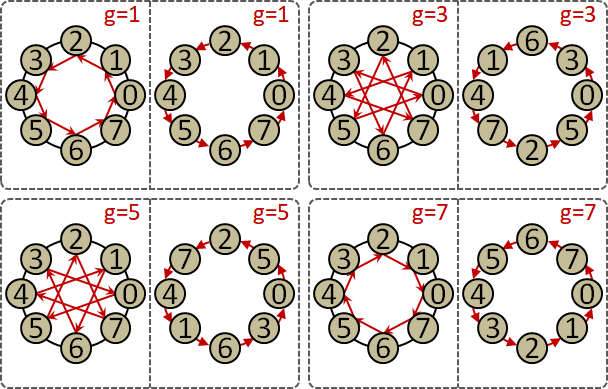Permutation Group

Permutation

「排列」可以看成是雙射函數，每個數字變成另一個數字。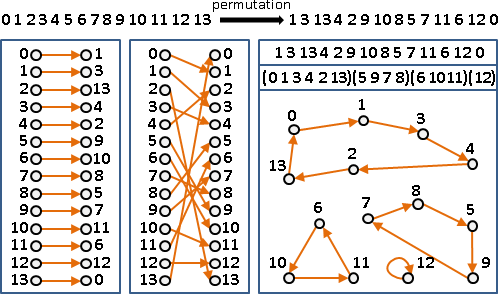UVa 11071 ICPC 6899

Orbit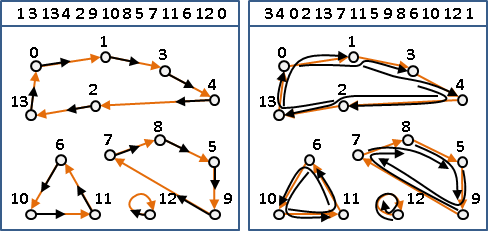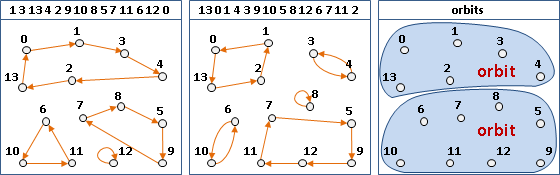Permutation Group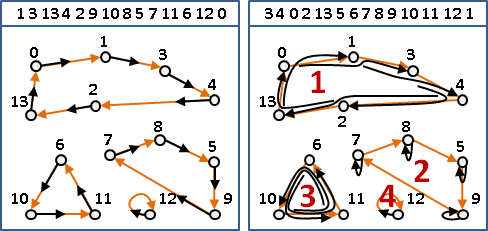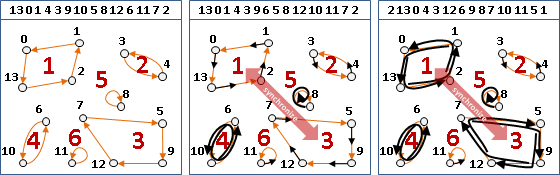Orbit–Stabilizer Theorem

```o(x) = { g‧x | g∈G }      從x可走到的點們。
orbit                    （一個環、一個連通分量）

s(x) = { g∈G | g‧x = x }  讓x走回原處的排列們。
stabilizer               （x的環走零步，其他環走隨意步）

f(g) = { x∈X | g‧x = x }  套用一個排列g，走回原處的點們。
fixed point              （某些環所走的步數，恰等於環的長度的倍數）
（某些連通分量所走的步法，恰回到原處）
```

```sum_all_x |s(x)| = sum_all_g |f(g)|
```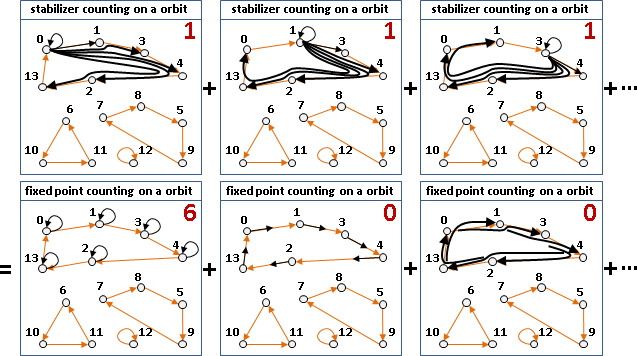Orbit Counting Theorem

```              |o(x)| |s(x)| =          #(g)   [orbit-stabilizer theorem]
sum_one_orbit |o(x)| |s(x)| =   |o(x)| #(g)   [repeat |o(x)| times]
|o(x)| sum_one_orbit |s(x)| =   |o(x)| #(g)   [constant]
sum_one_orbit |s(x)| =          #(g)
sum_all_orbit |s(x)| = #(orbit) #(g)
sum_all_x |s(x)| = #(orbit) #(g)
sum_all_g |f(g)| = #(orbit) #(g)   [Fubini's theorem]

sum_all_g |f(g)|
―――――――――――――――― = #(orbit)
#(g)
```

Pólya Counting Theorem

```                               #(cycles of g)
sum_all_g |f(g)|   sum_all_g  k
―――――――――――――――― = ―――――――――――――――――――――――――― = #(orbit)
#(g)                    #(g)
```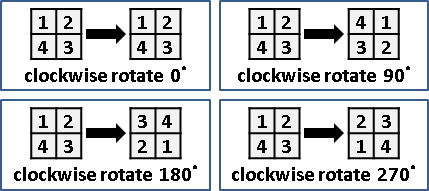```顏色k=2種                      循環節數量

|       g      | |f(g)| ||  cycle  | k^cycle
---- | -------------|--------||---------|---------
0° | (1)(2)(3)(4) |   16   ||    4    | 2^4 = 16
90° | (1234)       |    2   ||    1    | 2^1 = 2
180° | (13)(24)     |    4   ||    2    | 2^2 = 4
270° | (1432)       |    2   ||    1    | 2^1 = 2

orbit counting theorem: (16+2+4+2)/4 = 6
Pólya counting theorem: [(2^4)+(2^1)+(2^2)+(2^1)]/4 = 6
```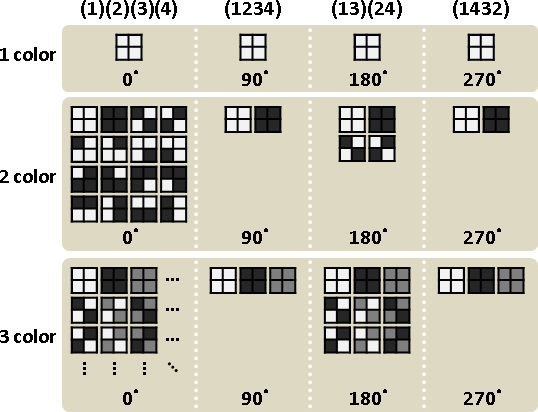UVa 10601 10733 11255 11540